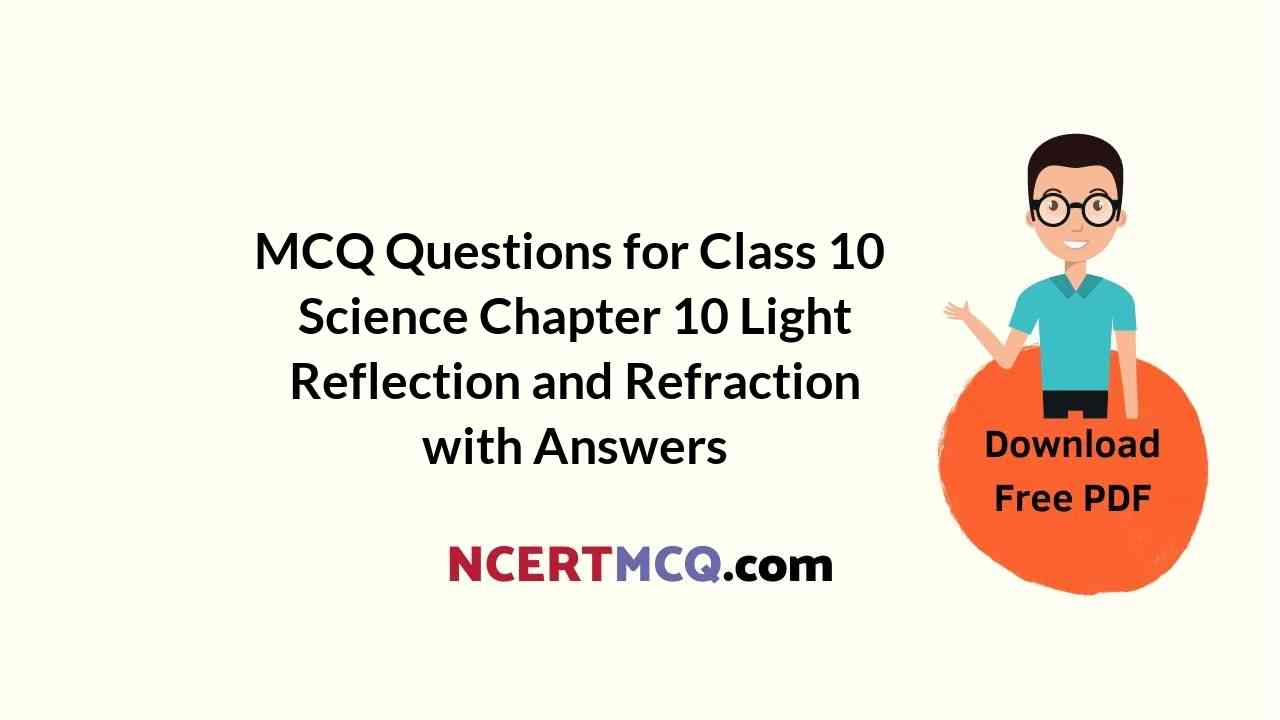Students can also read Online Education MCQ Questions for Class 10 Science Chapter 10 Light Reflection and Refraction Questions with Answers hope will definitely help for your board exams. https://ncertmcq.com/mcq-questions-for-class-10-science-with-answers/

## Online Education Light Reflection and Refraction Class 10 MCQs Questions with Answers

Light Reflection And Refraction Class 10 MCQ Question 1.
Beams of light are incident through the holes A and B and emerge out of box through the holes C and D respectively, as shown in the figure. Which of the following could be inside the box?(a) A rectangular glass slab
(b) A convex lens
(c) A concave less
(d) A prism

Light Class 10 MCQ Question 2.
A beam of light is incident through the holes on side A and emerges out of the holes on the other face of the box as shown in the figure. Which of the following could be inside the box?(a) Concave lens
(b) Rectangular, glass slab
(c) Prism
(d) Convex lens
(d) Convex lens

Explanation: In the given diagram, parallel rays converge at a point and emerge from face B. So, there will be a convex lens inside the box.Class 10 Light MCQ Question 3.
When an object is kept within the focus of a concave mirror, an enlarged image is formed behind the mirror. This image is:
(a) real
(b) inverted
(c) virtual and inverted
(d) virtual and erect

MCQ Of Light Class 10 Question 4.
A full length image of a distant tall building can definitely be seen by using:
(a) a concave mirror
(b) a convex mirror
(c) a plane mirror
(d) both concave as well as plane mirrorClass 10 Light Reflection And Refraction MCQ Question 5.
Your school laboratory has one large window. To find the focal length of a concave mirror using one of the walls as screen, the experiment may be performed:
(a) on the same wall as the window.
(b) on the wall adjacent to the window.
(c) on the wall opposite to the window.
(d) only on the table as per laboratory arrangement.
(d) only on the table as per laboratory arrangement.

Class 10 Science Chapter 10 MCQ Question 6.
The path of a ray of light coming from air passing through a rectangular glass slab is traced by four students shown as A, B, C and D in the figure. Which one of them is correct?MCQ On Light Class 10 Question 7.
In order to determine the focal length of a concave mirror by obtaining the Image of a distant object on screen, the position of the screen should be:
(a) parallel to the pLane of concave mirror
(b) perpendicular to the plane of concave mirror
(c) IncLined at an angle 600 to the plane of mirror
(d) in any direction with respect to the plane of concave mirror [Dikshaj
(a) parallel to the plane of concave mirror

Explanation: AD the refLected light rays converge and pass through the focus (F) of a concave mirror. Since the focus of a concave mirror is in front of the mirror, the screen has to be placed parallel in front of the mirror.

Light Reflection And Refraction MCQ Question 8.
A student carries out the experiment of tracing the path of a ray of Light through a rectangular glass slab for two different values of angLe of incidence ∠i = 300 and ∠i = 45° In the two coses the student is likely to observe the set of vaLues of angle of refraction and angLe of emergence as
(a) ∠r =30, ∠e = 20° and ∠r = 45’, ∠e = 28°
(b) ∠r =30°. ∠e = 30° and ∠r=45°, ∠e= 450
(c) ∠r =200, ∠e = 30° and ∠r = 28°, ∠e=45°
(d) ∠r =20°, ∠e = 20° and ∠r = 28°, ∠e = 28°
(c) ∠r=20° ∠e=30° and ∠r= 28 ∠e=45°

Related Theory:
The angle of refraction must be less than the angle of incidence. White angle of emergence is equal to angle of incidence.
for ∠i = 300. ∠r = 20° and ∠e = 30
for ∠i = 450e ∠r = 28° and ∠e = 45°

Reflection Of Light Class 10 MCQ Question 9.
If you focus a distant object of the shape using a concave mirror, the Image obtained must be of the shapeLight Chapter Class 10 MCQ Question 10.
In which of the following diagrams has the protractor (D) been correctly placed to measure the angle of incidence and the angle of emergence?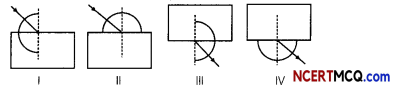a) I, III
(b) I, IV
(c) II, III
(d) II, IV
(a) I, III

Explanation: The angle of incidence is the angle between the incident ray and the normal at the point of incidence. The angle of emergence is the angLe between the emergent ray and the normal at the point of emergence.

Moreover, the protractor should always be placed in such a way that its base is always along the normal of the incident ray or the emergent ray.Ch 10 Science Class 10 MCQ Question 11.
An optical device has been given to a student and he determines its focal Length by focusing the image of the sun on a screen placed 24 cm from the device on the same side as the sun. Select the correct statement about the device.
(a) Convex mirror of Focal Length 12 cm
(b) Convex Lens of focal Length 24 cm
(c) Concave mirror of focal length 24 cm
(d) Convex tens of Focal Length 12 cm

MCQ Of Light Reflection And Refraction Class 10 Question 12.
A student is performing the experiment of determining the Focal Length of a given concave mirror by focussing a distant tree on a screen. Which one of the following kinds of images he is likely to obtain on the screen?(a) (I)
(c) (III)
(b) (II)
(d) (IV)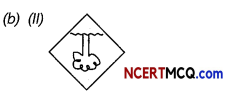Explanation: A concave mirror forms a real and inverted image of a distant object, such as tree, at its focus.

Class 10 Science Ch 10 MCQ Question 13.
A student has focussed on the screen a distant building using a convex lens. If he has selected a blue coloured building as object, select from the following options the one which gives the correct characteristics of the image formed on the screen.
(a) Virtual, erect, diminished and green shade
(b) Real, inverted, diminished and in violet shade
(c) Real, inverted, diminished and in blue shade
(d) Virtual, inverted, diminished and in blue shade
(c) Real, inverted, diminished and in blue shade

Explanation: As the student has focussed a distant blue coloured building on the screen using a convex lens, a real inverted and diminished image is formed at the principal focus of the convex lens.

As object is quite far, a diminished image will be formed at the principal focus.

The colour of image will be same as colour of the object because no change in colour takes place during refraction.

MCQ Questions On Light Class 10 Question 14.
Study the following four experimental setups by four students A, B, C and D showing the incident ray to trace the path of a ray of light through a glass slab. Which of these will get the best result?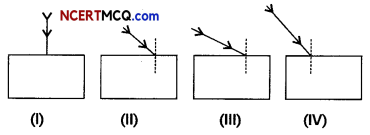(a) (I)
(b) (II)
(c) (III)
(d) (IV)

Light And Reflection Class 10 MCQ Question 15.
The focal length of the concave mirror in the following experimental set-up is:(d) 6.6 cm

Explanation: The least count of optical bench $$\frac{1}{5}$$cm = 0.2 cm
Position of the concave mirror = 12.8 cm
Position of the screen = 6.2 cm
Focal length of the concave mirror = distance of the screen from the pole of the concave mirror = 12.8 – 6.2 cm = 6.6 cm

Light Class 10 MCQ With Answers Question 16.
Three students A, B and C focussed a distant building on a screen with the help of a concave mirror. To determine focal length of the concave mirror they measured the distances as given below:
Student A: From mirror to the screen
Student B: From building to the screen
Student C: From building to the mirror
Who measured the focal length correctly:
(a) Only A
(b) Only B
(c) A and B
(d) B and C
(a) Only A

Explanation: A concave mirror forms a distinct image of a distant object at its focus. As student ‘A’ measures the distance between mirror to the screen to find the focal length, he measured the focal length correctly.

MCQ Questions For Class 10 Science Chapter 10 Question 17.
A student has traced the path of a ray of light through a glass slab as follows. If you are asked to label 1, 2, 3 and 4, the correct sequencing of labelling Zi, Ze, Zr and lateral displacement respectively is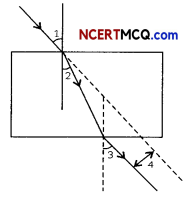(a) 2,1, 3, 4
(b) 1, 2, 3, 4
(c) 1, 3, 2, 4
(d) 1, 3, 4, 2
(c) 1, 3, 2, 4

Related Theory
Angle of emergence (e): The angle of the light coming out of a medium.
Lateral displacement: The perpendicular distance between the incident ray and the emergent ray.Light Reflection And Refraction Class 10 MCQ With Answers Question 18.
A student determines the focal length of a device X by focusing the image of a distant object on a screen placed 20 cm from the device on the same side as the object.
The device X is
(a) Concave lens of focal length 10 cm
(b) Convex lens of focal length 20 cm
(c) Concave mirror of focal length 10 cm
(d) Concave mirror of focal length 20 cm

Chapter 10 Science Class 10 MCQ Question 19.
A student obtained a sharp image of a candle flame placed at the distant end of the laboratory table on a screen using a concave mirror to determine its focal length. The teacher suggested him to focus a distant building about 1 km far from the laboratory, for getting more correct value of the focal length. In order to focus the distant building on the same screen the student should slightly move the:
(a) mirror away from the screen
(b) screen away from the mirror
(c) screen towards the mirror
(d) screen towards the building
(c) screen towards the mirror

Question 20.
A student obtains a sharp image of the distant window (W) of the school laboratory on the screen (S) using the given concave mirror (M) to determine its focal length. Which of the following distances should he measure to get the focal length of the mirror?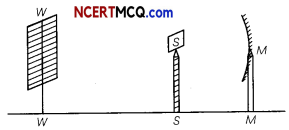(a) MW
(b) MS
(c) SW
(d) MW – MS

Question 21.
The image formed by a plane mirror is:
(a) virtual, behind the mirror and enlarged
(b) virtual, behind the mirror and of the same size as the object
(c) real, at the surface of the mirror and enlarged
(d) real, behind the mirror and of the same size as the object
(b) Virtual, behind the mirror and of the same size as the object

Question 22.
When object moves closer to convex lens, the image formed will be
(a) away from the lens on the other side of lens.
(b) towards the lens.
(c) away from the lens on the same side of an object.
(d) first towards and then away from the lens.

Question 23.
Which of the following ray diagrams is correct for the ray of light incident on a concave mirror as shown in figure?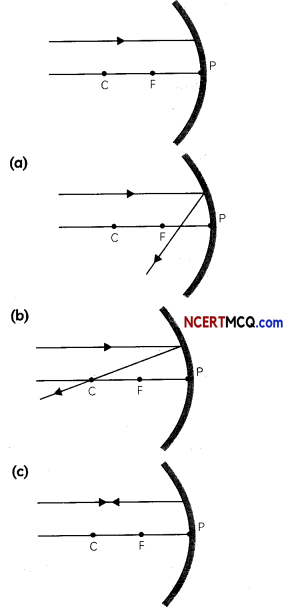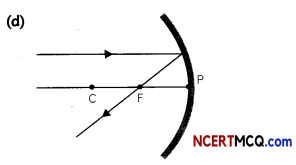Explanation: Ray of light parallel to the principal axis towards the mirror after reflection from the mirror will pass through the focus.

Question 24.
The refractive index of four substances P, Q, R and S are 1.50, 1.36, 1.77 and 1.31 respectively. The speed of light is the maximum in the substance:
(a) P
(b) Q
(c) R
(d) SQuestion 25.
Which one of the following materials cannot be used to make a lens?
(a) Water
(b) Glass
(c) Plastic
(d) Clay
(d) Clay

Explanation: Clay is opaque and does not let light to pass through it.

Question 26.
A small bulb is placed at the focal point of a converging lens. When the bulb is switched on, the lens produces:
(a) a convergent beam of light
(b) a divergent beam of light
(c) a parallel beam of light
(d) a patch of coloured light
(c) A parallel beam of light

Explanation: When an object is placed at the focus of a convex lens, the convex lens convert the diverging rays of light coming from the object into a parallel beam of light rays which form an image at infinity.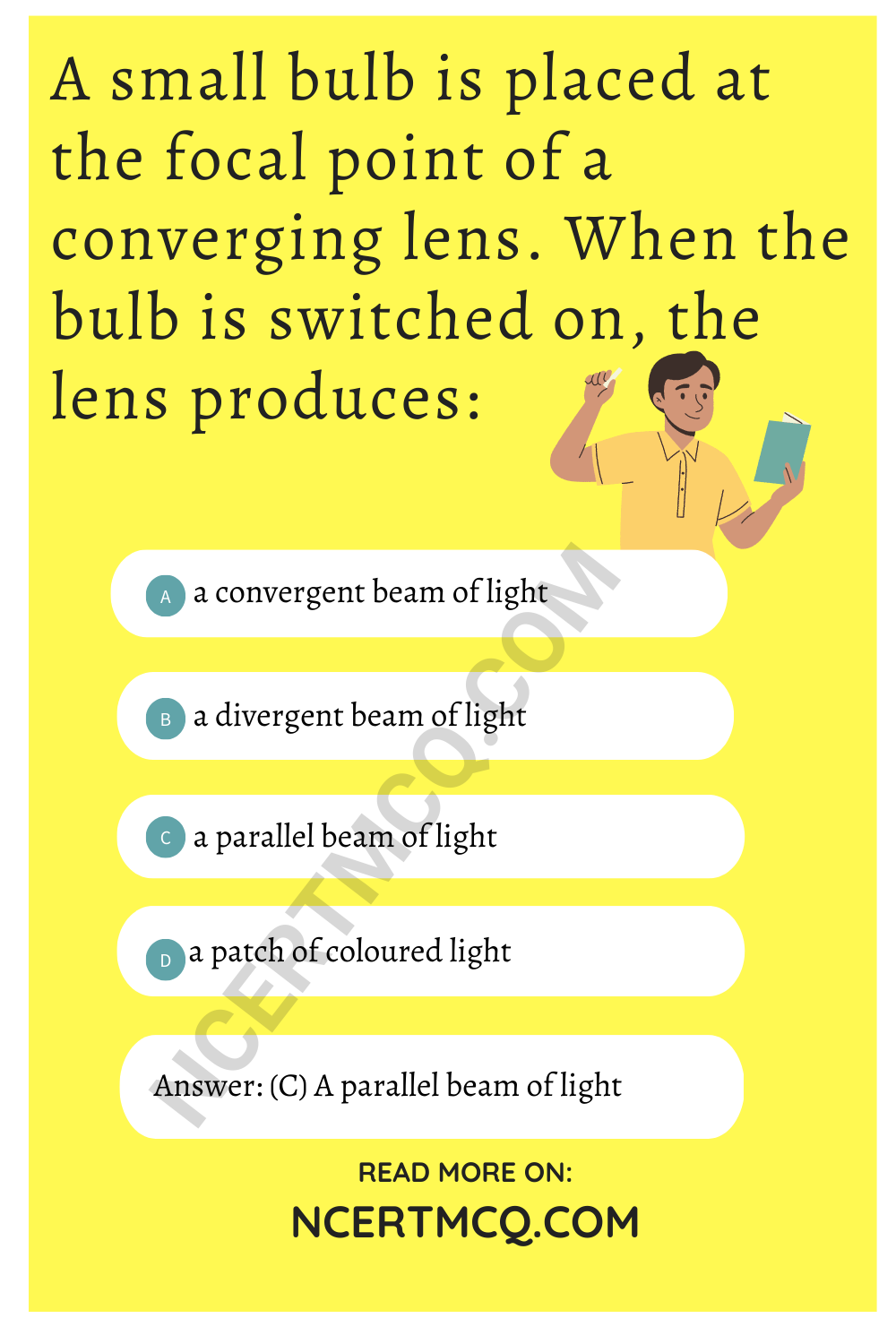Question 27.
In torches, search lights and headlights of vehicles, the bulb is placed:
(a) between the pole and the focus of the reflector
(b) very near to the focus of the reflector
(c) between the focus and centre of curvature of the reflector
(d) at the centre of curvature of the reflector

Question 28.
You are given water, mustard oil, glycerine and kerosene. In which of these media, a ray of light incident obliquely at some angle would bend the most? (Refractive index of water = 1.33, Mustard oil = 1.46, Glycerine = 1.473, Kerosene = 1.44)
(a) Kerosene
(b) Water
(c) Mustard oil
(d) Glycerine
(d) Glycerine

Explanation: Out of the given four materials, the refractive index of glycerine is highest. So, greatest deviation of incident light ray is observed in case of glycerine.

Related Theory
A substance having higher refractive index is optically denser than another substance having lower refractive index. We can conclude, the higher the refractive index of a substance, more it will change the direction of a beam of light passing through it.Question 29.
A student obtained a sharp inverted image of a distant tree on the screen placed behind a convex lens. He then removed the screen and tried to look through the lens in the direction of the object. He would now observe:
(a) a blurred image on the wall of the laboratory
(b) an erect image of the tree on the lens
(c) no image as the screen has been removed
(d) an inverted image of the tree at the focus of the lens

Question 30.
Magnification produced by a rear view mirror fitted in vehicles:
(a) is less than one
(b) is more than one
(c) is equal to one
(d) can be more than or less than one depending upon the position of the object in front of it.
(a) is less than one

Explanation: Convex mirror is used as a rear view mirror in vehicles. It forms virtual, erect and diminished images of the objects.

As magnification is defined as the ratio of the height of the image to the height of the object, hence, magnification produced by a rearview

Question 31.
You are provided four convex lenses of focal length 10 cm, 20 cm, 30 cm and 40 cm. Which out of the four lenses will converge light the most and the least?
Select the row containing the correct answer and reason: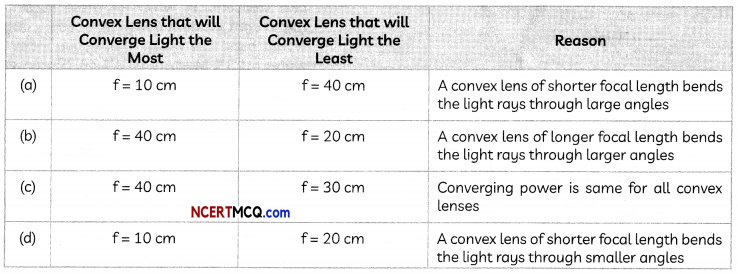Question 32.
A student-focussed the image of a candle flame on a white screen using a convex lens. He noted down the position of the candle screen and the lens as under
Position of candle = 26.0 cm
Position of convex lens = 50.0 cm
Position of the screen = 90.0 cm
Students noted down the values of object distance (u), image distance (v) and also calculated the focal length (f) of the convex lens used.

Select the row containing the correct values as per the sign convention:Question 33.
Select the correct statements from the statements given below regarding refraction of light when light is incident from a medium A having refractive index 1.85 on a glass slab having refractive index 1.50.
(I) Light will bend towards the normal in the glass slab.
(II) Emergent ray will be parallel to the refracted ray.
(III) Speed of light will be more in glass slab as compared to medium A.
(IV) Angle of refraction will be more than angle of incidence.
(a) Both (I) and (II)
(b) Both (II) and (III)
(c) Both (II) and (IV)
(d) Both (III) and (IV)
(d) Both (III) and (IV)

Explanation: As light is incident from the medium A having higher refractive index than glass, speed of light will be less in medium A as compared to glass. When light traveLs from a denser to a rarer medium, it bends away from the normal. Therefore, angle of refraction will be greater than the angle of incidence.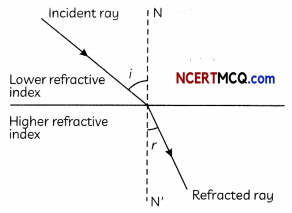Question 34.
Four statements are written below which are the general rules for drawing ray diagrams in case of lenses. Select the incorrect statements:
(I) A ray of light from the object, parallel to the principal axis, after refraction from a concave lens, passes through the principal focus on the other side of the lens.
(II) A ray of light appearing to meet at the principal focus of a convex lens, after refraction, will emerge parallel to the principal axis.
(III) A ray of light passing through a principal focus, after refraction from a convex lens, will emerge parallel to the principal axis.
(IV) A ray of light passing through the optical centre of a lens will emerge without any deviation.
(a) Both (I) and (II)
(b) Both (II) and (III)
(c) Both (I) and (IV)
(d) Both (III) and (IV)Question 35.
Given below are statements regarding sign conventions for reflection by spherical mirrors. Select the correct statements.
(I) All distances parallel to the principal axis are measured from the pole of the mirror.
(II) All the distances measured to the left of the origin (along – x-axis) are taken as positive while those measured to the right of the origin (along + x-axis) are taken as negative.
(III) Distances measured perpendicular to and above the principal axis (along + y-axis) are taken as positive.
(IV) Distances measured perpendicular to and above the principal axis (along +y-axis) are taken as negative.
(a) Both (I) and (II)
(b) Both (II) and (III)
(c) Both (I) and (III)
(d) Both (III) and (IV)

Question 36.
Refractive indices of turpentine oil, sapphire, water and crown glass are 1.47, 1.77, 1.33 and 1.52 respectively. In which of these media will a ray of light incident obliquely at the same angle from air would bend the least?
(a) Turpentine oil
(b) Sapphire
(c) Water
(d) Crown glass
(c) Water

Explanation: The speed of light in a medium is inversely proportional to the refractive index of the medium. And the amount of bending is also inversely proportional to the refractive index of the medium. As the refractive index of water is the least out of the given media, it is optically rarer as compared to the other media. So, light will bend the least in water.

Question 37.
Kaashvi performed an activity using a concave mirror of focal length 20 cm. She placed the object 30 cm in front of the mirror. Where is the image likely to be formed?
(a) At 10 cm behind the mirror
(b) At 10 cm in front of the mirror
(c) At 60 cm in front of the mirror
(d) At 60 cm behind the mirror

Question 38.
The angle between the incident and reflected rays is 90° as shown below:If the plane mirror is rotated by 10° about O in the anti-clockwise direction, then the angle between the incident and reflected rays will be:
(a) 55°
(b) 90°
(c) 100°
(d) 110°Question 39.
Two lenses, a convex lens of focal length 20 cm and a concave lens of focal length 25 cm, are held close to each other. The focal length of the combination of the lenses is:
(a) + 45 cm
(b) – 5 cm
(c) – 45 cm
(d) + 100 cm
(d) + 100 cm

Explanation: The power of combination of two lenses is P = P1 + P2, where P1 is the power of the convex lens of focal length 20 cm and P2 is power of the concave lens of focal length 25 cm.

As Power (in Dioptre) = P = $$\frac{1}{f(\text { inm })}$$
Therefore, $$\frac{1}{f_{1}}+\frac{1}{f_{2}}=+\frac{100}{20}-\frac{100}{25}$$ = +1
Thus, focal length of combination = +100 cm

Question 40.
Magnification produced by a rear view mirror fitted in vehicles:
(a) is less than one
(b) is more than one
(c) is equal to one
(d) can be more than or less than one depending upon the position of the object in front of it.
(a) is Less than one

Explanation: Convex mirror is used as a rear view mirror in vehicles. It forms virtual, erect and diminished images of the objects.

As magnification is defined as the ratio of the height of the image to the height of the object, hence, magnification produced by a rear view mirror, fitted in vehicles is less than one.

### Assertion Reasoning questions Class 10 Science Chapter 10

For the following questions, two statements are given one labelled Assertion (A) and the Other labelled Reason (R). Select the correct answers to these questions from the codes (a), (b), (c) and (d) as given below.
(a) Both (A) and (R) are true and (R) is the correct explanation of the (A).
(b) Both (A) and (R) are true, but (R) is not the correct explanation of the (A).
(c) (A) is true, but (R) is false.
(d) (A) is false, but (R) is true.

Question 41.
Assertion (A): A convex mirror is used as a rearview/driver’s mirror.
Reasoning (R): Convex mirrors have a wider field of view as they are curved outwards. They also give an erect, although diminished image. [Diksha]
(b) Both (A) and (R) are true but (R) is not correct explanation of the assertion. Explanation: Since the image formed is highly diminished in convex mirror, we can see the full image of far away objects, which is otherwise not possible in concave and plane mirrors.Question 42.
Assertion (A): The word AMBULANCE on the hospital vans is written in the form of its mirror image as AMBULANCE in inverted form.
Reason (R): The image formed in a plane mirror is same size of the object.
(b) Both (A) and (R) are true but (R) is not correct explanation of the assertion.

Explanation: The word AMBULANCE is written backwards (inverted) as when it is seen through a mirror in the vehicles ahead the drivers see it as AMBULANCE without inversion and give way to it on priority.

Question 43.
Assertion (A): Light travels faster in glass than in air.
Reason (R): Glass is denser than air.

Question 44.
Assertion (A): A convex lens has a real focus.
Reason (R): All light rays pass through the focus of a convex lens after refraction.
(c) (A) is true but (R) is false.

Explanation: A convex lens has a real focus as all the light rays parallel to the principal axis actuaLly pass through the focus of the convex lens after refraction. However, rays which are not parallel to the principal axis do not pass through the focus of the convex lens.

Question 45.
Assertion (A): A ray of light passing through the centre of curvature of a concave mirror is reflected back along the same path.
Reason (R): The incident ray, the reflected ray and the normal at the point of incidence lie on the same plane.
(b) Both (A) and (R) are true but (R) is not correct explanation of the assertion. Explanation: A ray of light passing through the centre of curvature of a concave mirror is reflected back along the same path because the rays are incident normally on the mirror. Therefore, angle of incidence = 0° and so, angle of reflection is also 0°. The law of reflection, namely, angle of incidence is equal to the angle of reflection is being followed here.Question 46.
Assertion (A): The power of a concave lens is negative.
Reason (R): A concave lens has a virtual focus.

(Competency Based Questions (CBQs))

Question 1.
Sushma went with her mother to purchase some gold jewelry for her sister’s marriage. The salesman asked them to check the hallmark and gold purity to ascertain the genuineness of the gold article. As the hallmark was too small to be seen by the naked eye, the salesman gave them a magnifying glass to see the hallmark.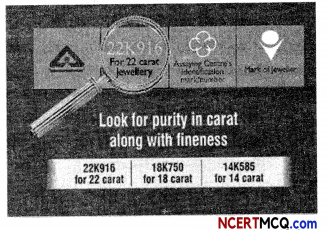Which type of lens is used as a magnifying glass and why?
A convex lens is used as a magnifying glass as it forms an enlarged or magnified image of an object when it is held close to the object to be seen.

Question 2.
Analyse the following observation table showing variation of image-distance (v) with object-distance (u) in case of a convex lens and answer the questions that follow without doing any calculations:

 S.NO Object-Distance u (cm) Image-Distance v (cm) 1. -60 – +12 2. -30 – +15 3. -20 – +20 4. -15 – +30 5. -12 – +60 6. -9 – +90

(A) What is the focal length of the convex lens? State reason for your answer.
From S.No. 3, we can say that the radius of curvature of the lens is 20 cm because when an object is placed at the centre of the curvature of a convex lens its image is formed on the other side of the lens at the same distance from the lens. And, we also know that focal length is half of the radius of curvature. Thus, focal length of the lens is + 10 cm.

(B) For what object-distance (u) is the corresponding image-distance (v) not correct? How did you arrive at this conclusion?
S.No. 6 is not correct as for this observation the object distance is between focus and pole, and for such cases the image formed is always virtual but in this case, a real image is forming as the image distance is positive.

(C) A concave mirror gives real, inverted and same size image if the object is placed
(a) At focus
(b) At infinity
(c) At C i.e. centre of curvature
(d) Beyond centre of curvature
(c) At C i.e. centre of curvature

(D) Focal length of plane mirror is:
(a) At infinity
(b) Zero
(c) Negative
(d) None of theseQuestion 3.
While sipping some Lemonade with a straw, Ronit observed that the straw Looked bent when seen from the side. He could guess that it was due to the bending of Light or refraction.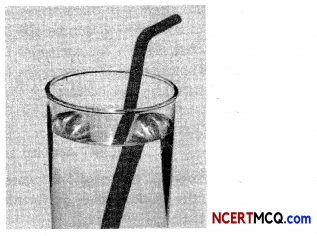The figure shows a ray of Light as it travels from medium A to medium B. The refractive index of the medium B relative to medium A is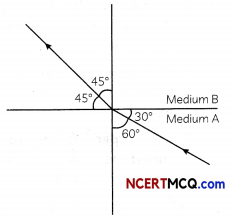(a) $$\frac{\sqrt{3}}{\sqrt{2}}$$
(b) $$\frac{\sqrt{2}}{\sqrt{3}}$$
(c) $$\frac{1}{\sqrt{2}}$$
(d) $$\sqrt{2}$$
(a) $$\frac{\sqrt{3}}{\sqrt{2}}$$

Explanation: Here, angle of incidence, i = 60° Angle of refraction, r = 45°
The refraction index of the medium B relative to medium A,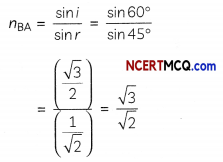Question 4.
Analyze the following table showing refractive indices of four media A, B, C and D and answer the questions that follow:
The refractive indices of four media A, B, C and D are given in the following table: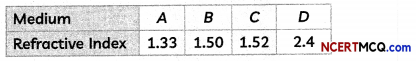(A) If light, travels from one medium to another in which case the change in speed will be minimum.
Minimum change is seen as light moves between 1.50 and 1.52, i.e. B and C.

(B) If Light, travels from one medium to another, in which case the change in speed will be maximum.
Maximum change when light moves between 1.33 and 2.40, i.e. A and D.

(C) A Light ray enters from medium A to medium B as shown in the figure. The refractive index of medium B relative to A will be: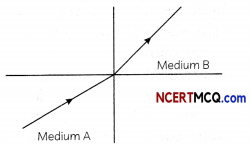(a) Greater than unity
(b) Less than unity
(c) Equal to unity
(d) Zero
(a) Greater than unity

Explanation: Since light rays in medium B goes towards normal. So it has greater refractive index and lesser velocity, of light with respect to medium A. So refractive index of medium B with respect to medium A is greater than unity.

(D) The laws of reflection hold good for
(a) plane mirror only
(b) concave mirror only
(c) convex mirror only
(d) all mirrors irrespective of their shapeQuestion 5.
Parag along with his friends were experimenting with a concave mirror. They tried to focus the sun’s rays at a piece of paper and observed that the paper started to burn after some time.Rays from the sun converge at a point 15 cm in front of a concave mirror. Where should an object be placed so that the size of its image is equal to the size of the object?
(a) 15 cm in front of the mirror.
(b) 30 cm in front of the mirror.
(c) Between 15 cm and 30 cm in front of the mirror.
(d) More than 30 cm in front of the mirror.
(b) 30 cm in front of the mirror.

Explanation: The sun is at an infinite distance from the surface of the Earth. Now, light rays from the sun rays i.e., from infinity, are parallel to the principal axis. After reflection from the concave mirror, the light rays will converge at focus. It is given that the sun rays converge at 15 cm.

So, the focal length of the mirror, f = 15 cm. We know that in case of a concave mirror, the same size, real and inverted image is formed when object is placed at 2F or the centre of curvature.
Hence, in this case, object must be place at 2F i.e., 2 x 15 = 30 cm.

Question 6.
First, hold a convex lens in your hand and direct it towards the Sun. Focus the light from the Sun on a sheet of paper. Obtain a sharp bright image of the Sun. Hold the paper and the lens in the same position for a while. Keep observing the paper. While performing this activity, do not look at the Sun directly or through a lens or otherwise you may damage your eyes if you do so.
Next, take a convex lens. Find its approximate focal length in a way described in Activity above. Draw five parallel straight lines, using chalk, on a long Table such that the distance between the successive lines is equal to the focal length of the lens. Place the lens on a lens stand. Place it on the central line such that the optical centre of the lens lies just over the line. Place a burning candle, far beyond 2F1 – to the left. Obtain a clear sharp image on a screen on the opposite side of the lens. Repeat this Activity by placing object just behind 2F1, between F1 and 2F1, F1 at 1, between F1 and O. Note down and tabulate your observations.
(A) Following observations were recorded by the student after performing the first activity:
(I) A large and bright image of the sun is obtained on a paper when light from sun is focused.
(II) A sharp and bright image of the sun is obtained on a paper when light from sun is focused.
(III) The paper begins to burn immediately when both the paper and lens are held in the same position.
(IV) The paper begins to burn after some time when both the paper and lens are held in the same position.
Select the incorrect observations:
(a) Both (II) and (III)
(b) Both (II) and (IV)
(c) Both (I) and (III)
(d) Both (I) and (IV)
(c) Both (I) and (III)

Explanation: Convex lens is a converging lens. So, it converges the light rays from the sun and a sharp bright image of the sun is obtained on a paper as sun is very very far from us. When the lens and paper are held in the same position for some time, it is observed that the paper begins to burn after some time as all the sun’s rays converge at the bright spot, which is the focus of the lens, causing the paper to burn.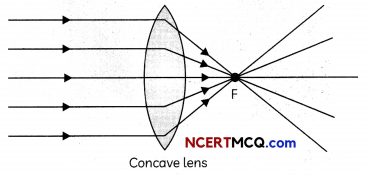(B) Two students performed the second activity for which as per directions mentioned above.
They drew five lines and marked the line numbers from 1 to 5 above the lines.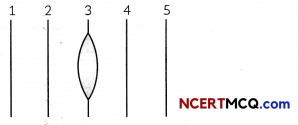The teacher asked the students to keep a record of the lines marked. The students tabulated the record as given below:

 First Student Second Student (a) Line numbers 1 and 2 are 2F1 and F1 respectively Line number 1 and 5 are F1 and 2F1 respectively (b) Line numbers 4 and 5 are F2 and 2F2 respectively Line number 2 and 4 are and F2 respectively (c) The central line (line number 3) contains the focus of the lens The central line (line number 3) contains the optical center (d)Distance between two successive lines is equal to twice the focal length of the lens Distance between two successive lines is equal to the focal length of the lens

Select the row containing the correct information by both the students.
(b) First student: Line numbers 4 and 5 are F2 and 2F2 respectively; Second student: Line number 2 and 4 are F1 and F2 respectively

Explanation: The lens has been placed on the central line which contains the optical centre of the lens. Lines 1 and 2 are 2F1 and F1 respectively and lines 4 and 5 are F2 and 2F2 respectively. Moreover, the distance between successive lines is equal to the focal length of the lens, which is OF1.(C) Select the incorrect statement. In the first activity:
(a) A real image of the sun is obtained on the paper
(b) A virtual image of the sun is obtained on the paper.
(c) The convex lens converges the sun’s rays at the bright spot.
(d) The approximate focal length of the convex lens is the distance between the lens and the paper.
(b) A virtual image of the sun is obtained on the paper.

Explanation: Convex lens is a converging lens and converges the parallel rays of light coming from the sun at the bright spot formed on the paper. The image formed is therefore real. Further, the distance between the lens and the paper gives the approximate value of the focal length of the lens.

(D) The image of a burning candle placed beyond 2F1 to the left is:
(a) Real, inverted, diminished and formed on a screen placed at the opposite side of the lens.
(b) Real, inverted and enlarged and formed on the same side as the candle.
(c) Virtual, erect, diminished and formed on the same side as the candle.
(d) Real, inverted, enlarged and formed on a screen placed at the opposite side of the lens.

(E) As the candle is brought closer to the focus F-L from the left:
(a) Image becomes smaller in size
(b) Image size first becomes smaller and then enlarges
(c) Image size first becomes larger and then diminishes.
(d) Image becomes Larger in size.Question 7.
When Aiysha visited her ENT specialist to consult the doctor as she had some problem in her ear, she saw a circular mirror worn by the doctor around the forehead. She wanted to know the purpose served by the mirror. So, she came home and tried to find out the type of mirror and its applications.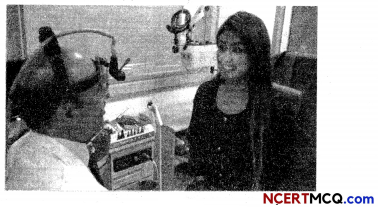Write two different uses of concave mirrors.
Uses of concave mirrors:
(1) It is used in torches, headlights of vehicles and search-lights to get powerful parallel beams of light.
(2) It is used as a shaving mirror to see a larger image of the face when held close to the face.
(3) It is used by dentists and ENT specialists to see larger images of teeth and inner parts of ear and throat.
(4) Large concave mirrors or parabolic mirrors are used in solar furnaces to concentrate sunlight.

Question 8.
There are numerous uses of concave mirrors in daily life. It is used in the aircraft landing to guide the airplane, it is used as a torch to reflect the light rays, it is used during shaving to get an erect and enlarged image of the face, etc.
A student had a concave mirror with him and wanted to know its focal length. So, he performed an activity to find out the focal length of the concave mirror.(A) The radius of curvature of the concave mirror used by the student in the above activity is:
(a) 60 cm
(b) 40 cm
(c) 30 cm
(d) 10 cm
(a) 60 cm

Explanation: The focal length of the mirror is the distance between the pole of the mirror and the screen where the clear image of the distant object is formed. As the mirror stand is at 10 cm and screen is at 40 cm, therefore, focal length = 40-10 = 30 cm, as the image of a distant object is formed by a concave mirror at its focus. Radius of curvature = 2f = 60 cm.

(B) Select the correct observation :
(I) Image of the distant tree is formed by the concave mirror at its focus.
(II) Image of the distant tree is formed by the concave mirror at its centre of curvature.
(III) Image is virtual and laterally inverted
(IV) Image is real and inverted
(a) Both (I) and (III)
(b) Both (II) and (III)
(c) Both (I) and (IV)
(d) Both (II) and (IV)
(c) Both (I) and (IV)

Explanation: A concave mirror forms a real and inverted image of a distant object at its focus as shown in the figure above. Image is real as the rays of light intersect at the focus after reflection from the concave mirror.

(C) It is desired to obtain an erect image of an object, using concave mirror of radius of curvature 40 cm. Two students gave their ideas in a tabular form as given below:

 First Student Second Student (a) Range of object distance should be from 0 cm to less than 20 cm Range of object distance should be from 0 cm to less than 40 cm (b) Image can be obtained at a screen placed at the position of formation of image Image cannot be obtained on a screen placed at the position of formation of image (c) Image will be larger than the object Image will be of same size as the object (d) Image will be formed behind the mirror. Image will be formed behind the mirror.

The row containing the correct information by both the students is:

(D) In the situation mentioned in question (C) above, if the object is kept at 40 cm, the position and nature of image formed will be:
(a) Real, inverted and enlarged image will be formed 40 cm in front of the mirror.
(b) Real and inverted image will be formed 40 cm in front of the mirror of same size as object.
(c) Virtual, erect and enlarged image will be formed 40 cm behind the mirror.
(d) Virtual and erect image will be formed 40 cm behind the mirror of same size as object.

(E) The magnification produced by a concave mirror when object is placed between Pole and Focus is:
(a) m = +1 (b) m = -1
(c) m > +1 (d) m > -1
(c) m > + 1

Explanation: The magnification produced by a mirror is the ratio of height of image to the height of object. As the image formed by a concave mirror when object is placed between pole and focus is virtual, erect and magnified, m > + 1.Question 9.
While brushing her teeth one morning, Reema noticed that while she was holding the tooth brush in her left hand, it was just the opposite in her image formed by the plane mirror. She was amused but knew why it was so!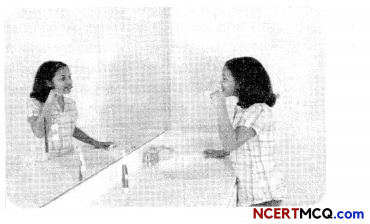What is the name of the phenomenon in which the right side of an object appears to be the left side of the image in a plane mirror?
Lateral inversion is the phenomenon in which the right side of an object appears to be the left side in its image in a plane mirror.

Question 10.
One of the most common uses for the convex mirror is the passenger-side mirror on your car. Convex mirrors are also often found in the hallway of various buildings including hospitals, hotels, schools, stores and apartment buildings.
Usually, these mirrors are mounted to a wall or ceiling at points where hallways cross each other or make a sharp turn. This eliminates blind spots and provides people with a good overview of their surroundings. The convex mirror is also used to provide safety for motorists on roads, driveways and in alleys where there is a lack of visibility.A convex mirror is also a simple way of improving the safety in your warehouse or production environment. In a work environment or warehouse, convex mirrors can be placed at crossings or blind spots to enable workers to see approaching forklifts, other vehicles or approaching colleagues. This provides your employees with the necessary overview of their work environment and therefore increases the safety in your workplace.

Another use of the convex mirror in this work environment is during the production process, such as on the conveyor belt to view your product from different angles. This can increase the quality of your products by becoming aware of any faults in the production and increase the efficiency of the production process by eliminating the necessity to check your products by picking them up from the conveyor belt.
(A) Select the characteristics of convex mirror due to which it is used as rear view mirrors.
(I) Convex mirror always forms a virtual and erect image
(II) Convex mirrors may form real or virtual image depending upon the position of object
(III) Convex mirrors provide a wider field of view as they are curved outwards.
(IV) Images formed by convex mirrors are usually larger than the object.
(a) Both (I) and (III)
(b) Both (II) and (III)
(c) Both (I) and (IV)
(d) Both (III) and (IV)
(a) Both (I) and (III)

Explanation: Convex mirrors are used as rearview mirrors in cars because they always form virtual and erect image and provide a wider field of view as they are curved outwards.(B) As the object is moved away from the focus of the convex mirror:
(a) size of image does not change
(b) size of image increase
(c) size of image decreases
(d) cannot be ascertained as size of image depends upon the focal length of the convex mirror.

(C) The magnification produced by a convex mirror is always:
(a) Equal to +1
(b) Equal to -1
(c) Greater than +1
(d) Smaller than +1

(D) The image formed by a convex mirror of focal length 30 cm is a quarter of the object. The object distance and image distance, along with the sign convention, are tabulated below. Select the row containing the correct values of object and image distance:

 Object Distance (u) Image Distance (v) (a) – 90 cm + 22.5 cm (b) – 22.5 cm + 90 cm (c) – 90 cm – 22.5 cm (d) – 90 cm + 90 cm

(a) Object Distance : -90 cm ; Image Distance: +22.5cm

Explanation: it is given that focal Length, f = + 30 cm and size of image = VA times size of object.(E) Consider an object 7.0 cm in length which is placed at a distance of 20 cm in front of a convex mirror of radius of curvature 30 cm. The size and position of image formed is:
(a) size of image = -3 cm, position of image = + $$\frac{60}{7}$$ cm
(b) size of image = +3 cm, position of image = + $$\frac{60}{7}$$ cm
(c) size of image = +3 cm, position of image = + $$\frac{7}{60}$$ cm
(d) size of image = -3 cm, position of image = + $$\frac{7}{60}$$ cm
(b) size of image = + 3 cm, position of image = + $$\frac{60}{7}$$ cm

Explanation: Here, height of object = h1 = 7 cm; u = – 20 cm, f= + 30/2cm = + 15cm.
Applying the mirror formula, we get.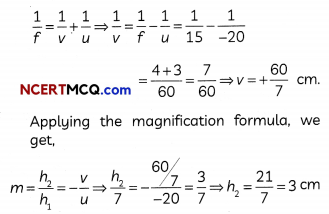Question 11.
When we look down into a pool of water from above, the pool looks less deep than it really is. Similarly, an object kept at the bottom of a pond or pool of water appears to be raised.

This great blue heron finally catches a meal after seeking the waters. To be a successful hunter, the heron must use the refraction principle to determine where the fish are located before making a strike. The heron can accurately determine where to strike by recognizing that the fish are located at a spot that is different from what its eyes tell it.(A) The apparent depth of the fish in water
(a) Less than the actuaL depth because Light travels from a rarer medium to denser medium.
(b) Less than the actuaL depth because Light travels from a denser medium to rarer medium.
(c) More than the actual. depth because of total internat reflection of Light.
(d) More than the actual depth because Light travels from a denser medium to rarer medium.
(b) Less than the actual depth because light travels from a denser medium to rarer medium.

Explanation: As light travels from a denser medium (water) to a rarer medium (air), the light rays undergo refraction and bend away from the normal. To an observer, the position of fish in water appears to be raised as shown in the figure above. Therefore, the apparent depth of the fish in water is less than the actual depth due to refraction of light.

(B) A student was thinking what would happen if the medium is changed from water (refractive index of water = 1.33) to a new medium A having refractive index 1.5. He listed all possibilities in a tabular form.

Select the row containing the correct observation and reason.

 Observation Reason (a) Speed of light will be more in the new medium A Speed of light in a medium is directly proportional to the refractive index of the medium. (b) Light rays will bend more in water as compared to A Bending of light is more in a rarer medium as compared to a denser medium (c) Apparent depth of fish will be more in water as compared to medium A Light rays will bend when coming from medium A. (d) Apparent depth of fish will be less in water as compared to medium Light rays will bend less when coming from medium A.

(c) Observation – Apparent depth of fish will be more in water as compared to medium A;
Reason: Light rays will bend when coming from medium A.

Explanation: When the fish is kept in medium A, light rays will bend more as compared to water because of which fish will appear to be raised more in A as compared to water.

(C) Select the incorrect statement from the statements below regarding refractive index of a medium:
(a) The refractive index can be (inked to the relative speed of propagation of
tight in different media.
(b) Light propagates with different speeds in different media.
(c) Refractive index of air is approximately equal to 1 as speed of tight is fastest In vacuum.
(d) The refractive index of glass is less than that of water as speed of tight in glass is 2 × 108 m/s and in water is 2.25 × 108 m/s.

(D) Which of the following are not examples of the phenomena of refraction of light?
(I) Spectacles worn by people to correct their defect of vision
(II) Formation of rainbow
(III) Solar furnaces
(IV) SatelLite dishes
(a) Both (I) and (II)
(b) Both (II) and (III)
(c) Both (III) and (IV)
(d) Both (II) and (IV)
(c) Both (III) and (IV)

Explanation: Solar furnaces and satellite dishes use parabolic or concave mirrors and make use of the phenomenon of reflection of light, whereas spectacles worn by people having defective vision and formation of rainbow are based on the phenomenon of refraction of light.

(E) A ray of light continues moving along the same path while passing through air- water interface. The angle of refraction for the ray is:
(a) 0°
(b) 45°
(c) 90°
(d) Greater than 90°Question 12.
Sumati wanted to see the stars of the night sky. She knows that she needs a telescope to see those distant stars. She finds out that the telescopes, which are made of lenses, are called refracting telescopes and the ones which are made of mirrors are called reflecting telescopes.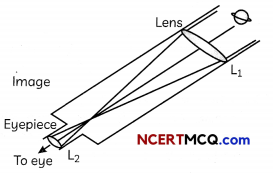So, she decided to make a refracting telescope. She bought two lenses, L1 and L2 out of which L1 was bigger and L2 was smaller. The larger lens gathers and bends the light, while the smaller lens magnifies the image. Big, thick lenses are more powerful. So to see far away, she needed a big powerful lens. Unfortunately, she realized that a big lens is very heavy.

Heavy lenses are hard to make and difficult to hold in the right place. Also since the light is passing through the lens, the surface of the lens has to be extremely smooth. Any flaws in the lens will change the image. It would be like looking through a dirty window.
(A) Based on the diagram shown, what kind of lenses would Sumati need to make the telescope?
(a) Concave lenses
(b) Convex lenses
(c) Bifocal lenses
(d) Flat lenses
(a) Concave lenses

(B) If the powers of the lenses L1 and L2 are in the ratio of 4 : 1, what would be the ratio of the focal length of L1 and L2?
(a) 4 :1
(b) 1: 4
(c) 2:1
(d) 1:1

(C) What is the formula for magnification obtained with a lens?
(a) Ratio of height of image to height of object
(b) Double the focal length
(c) Inverse of the radius of curvature
(d) Inverse of the object distance

(D) Sumati did some preliminary experiment with the lenses and found out that the magnification of the eyepiece (L2) is 3. If in her experiment with L2 she found an image at 24 cm from the lens, at what distance did she put the object?
(a) 72 cm
(b) 12 cm
(c) 8 cm
(d) 6 cm
(c) 8 cm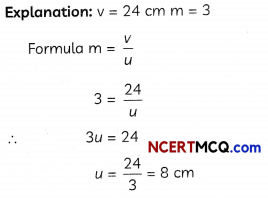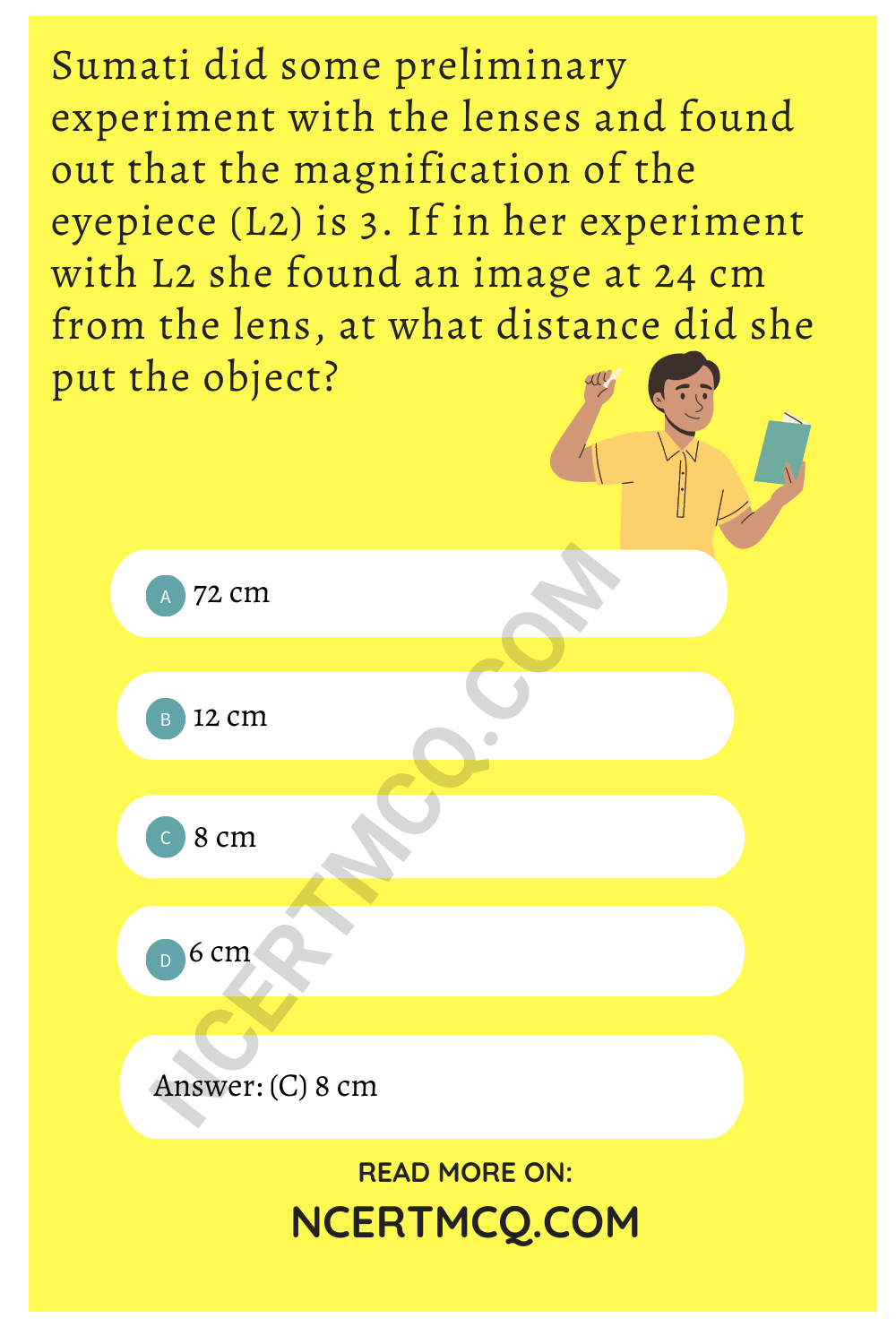(E) Sumati bought not-so-thick lenses for the telescope and polished them. What advantages, if any, would she have with her choice of lenses?
(a) She will not have any advantage as even thicker lenses would give clearer images.
(b) Thicker lenses would have made the telescope easier to handle.
(c) Not-so-thick lenses would not make the telescope very heavy and also allow considerable amount of light to pass through them.
(d) Not-so-thick lenses will give her more magnification.
(c) Not-so-thick lenses would not make the telescope very heavy and they will also allow considerable amount of light to pass through them.

Question 1.
Which type of mirror should a student use if he wants to project the image of a candle flame on the walls of the school laboratory?Question 2.
If a magnification of -1 is to be obtained using a concave mirror of focal length 6 cm, then what should be the position of the object?
Magnification of -1 means that the image is real and size of image is equal to the size of the object. This is possible when the object is placed at C of a concave mirror. Therefore, position of object = C = 2f = 12 cm in front of the concave mirror.

Question 3.
A man stands 10 m away in front of a large plane mirror. How far must he walk before he is 5 m away from his image?
As the distance between the man and the mirror is 10 m, the distance between man and his image is 10 + 10 or 20 m, as image distance and object distance are equal. Distance between the man and his image is 5 m when the man is 2.5 m away from the mirror. Therefore, the man has to walk 10 m- 2.5m = 7.5 m towards the mirror.

Question 4.
Find the focal length of a convex mirror whose radius of curvature is 32 cm.

Question 5.
Observe the given figure. What is the angle of reflection?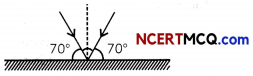The angle of reflection is the angle between the normal and the reflected ray.
Therefore: 900 70° = 200

Question 6.
An object O is placed in front of a plane mirror as shown beLow.At which point is the image of the object ‘O’ formed?
At point R, the image of object ‘O’ is formed.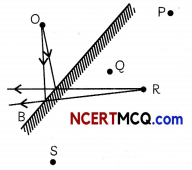Question 7.
Define power of a lens.Question 8.
Both a spherical mirror and a thin spherical lens have a focal length of (-) 15 cm. What type of mirror and lens are these?
Both are concave
Alternative answer that should be given credit: Plano-concave lens

Explanation: Focal length of a concave mirror is the distnace of principal focus F of the mirror from the pole P of the mirror. In concave lens – Focal length is the distnace of principal focus of the lens from the optical centre C. All the distances parallel to the principal axis of the spherical mirror, are measured from the pole of the mirror and in lens this distance is measured from the optical centre of the lens.

In mirror or lens the distances measured in a direction opposite to the direction of incident light are taken as negative.

Concave mirror:Concave lens: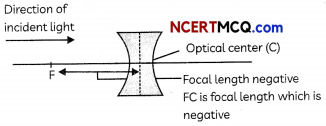Question 9.
The image formed by a concave mirror is observed to be real, inverted and larger than the object. Where is the object placed?